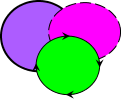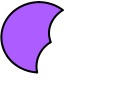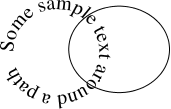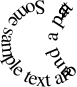Previous: Path Intersection  Up: Selecting and Editing Objects  Next: Separating a Text-Path into a Text Area and Path

# Path Subtraction

Multiple shapes can be combined into a single shape by performing a subtraction on all the selected shapes using the menu item Transform->Subtract Paths. At least two shapes must be selected to perform this function. The new shape is the backmost selected shape with the other selected shapes subtracted from it. Any patterns in the selection will be converted to full paths.

For example, in Figure 8.40(a), there are three overlapping paths. In Figure 8.40(b) the paths have been replaced by a single path created using the path subtraction function.

The new path will be a text-path if the backmost selected object was a text-path and the text will adjust to fit the new underlying path. For example, in Figure 8.41(a), there is a text-path and a path. The text-path is the backmost path. In Figure 8.41(b), the two objects have been replaced by a single text-path using the path subtraction function.(a) (b)
Figure 8.40: Path subtraction function: (a) original paths (the rear path has an orchid fill colour and round join style); (b) the three paths in (a) have been replaced by a single path using the path subtraction function.(a) (b)
Figure 8.41: Subtracting from a text-path: (a) original text-path and path; (b) the path has been subtracted from the underlying path of the text-path.